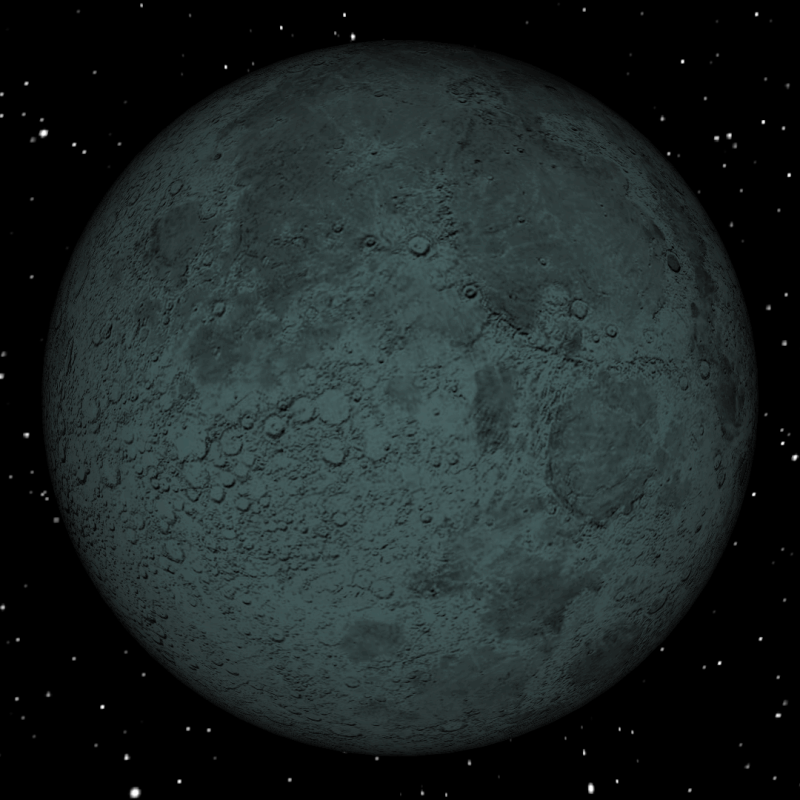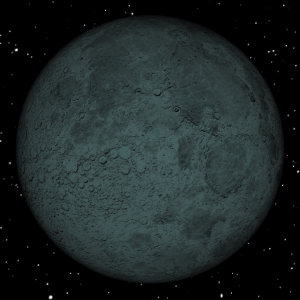# Numerology Of 1 Synchronicity With Five New Moons

(Exercising Your Mind) From April 18th 2015 until August 14th 2015, any person born on September 17th of any year, will have a Numeral 1 Personal Day Vibration that falls on the same day as there will be for a New Moon!

This means that for 5 New Moons in a row, the birthday of 9-17 will have the vibration of Leadership, Creativity, and Starting New Things, synchronized for powerful New Beginnings!### Sample Numerology Calculations

September 17th 1977 = 9 + 1+ 7 + 1 + 9 + 7 + 7 = 41 or

4 + 1 = 5. Therefore, according to Numerology, the birthday just calculated indicates that a person with this birth-date is influenced by the Destiny of the vibrations under the Numeral 5.

A person’s Destiny Numeral is one of the primary vibrations that stays with an individual for their whole life.

Alternative calculations get you the same Numeral / Number

9 + 17 = 26 and 2 + 6 = 8

1 + 9 + 7 + 7 = 24 and 2 + 4 = 6

You can add 26 + 24 = 50 and 5 + 0 = 5 or,

add 8 + 6 = 14 and 1 + 4 = 5

Another major influence is the person’s name, which is calculated by the numerals that match up with the letters in their name. (We will explore that another time)

Related: New Month, New Moon, Numerology

Using Numerology to determine the best times for certain personal actions, has proven to be quite successful for me through demonstrations I have made in my life.

There is an amazing PodCast that I recorded that will initiate you to this proof called:

Money, Magic, MetaPhysics, and Music

Calculations That Demonstrate The Numeral 1 And New Moon Synchronicity

The Science of Numerology teaches that each person’s individual year vibration, resonates from the last birthday that they had until their next birthday.

So for a person who was born on September 17th, at the time of this writing (5-22-2015), their last birthday was September 17th, 2014.

So, we calculate their personal year vibration by using the numeral calculated for that year.

Remember that September 17 is equal to: 9 + 1 + 7 = 17 and 1 + 7 = 8

2014 is equal to: 2 + 0 + 1 + 4 = 7

Adding 7 + 8 = 15 and 1 + 5 = 6

Therefore, the Personal Year Vibration for September 17th between their birthday in 2014 until their birthday in 2015 is equal to 6.

### September 17th and April 2015

To get their vibration for April, which is the 4th month, you simply add 6 + 4 = 10 and 1 + 0 = 1

This means that the Personal Month Vibration is equal to 1 for the September 17th birthday.

Then, adding the date of the New Moon in April, which is the 18th to the month vibration to get the Personal Day Vibration you end up with 1.

Because the month equals 1 + 18 = 19 and 1 + 9 = 10 and 1 + 0 = 1.

### September 17th and May 2015

To get their vibration for May, which is the 5th month, you simply add 6 + 5 = 11 and 1 + 1 = 2

This means that the Personal Month Vibration is equal to 2 for the September 17th birthday.

Then, adding the date of the New Moon in May, which is the 17th to the month vibration to get the Personal Day Vibration you end up with 1.

Because the month equals 2 + 17 = 19 and 1 + 9 = 10 and 1 + 0 = 1.

### September 17th and June 2015

To get their vibration for June, which is the 6th month, you simply add 6 + 6 = 12 and 1 + 2 = 3

This means that the Personal Month Vibration is equal to 3 for the September 17th birthday.

Then, adding the date of the New Moon in June, which is the 16th to the month vibration to get the Personal Day Vibration you end up with 1.

Because the month equals 3 + 16 = 19 and 1 + 9 = 10 and 1 + 0 = 1.

### September 17th and July 2015

To get their vibration for July, which is the 7th month, you simply add 6 + 7 = 13 and 1 + 3 = 4

This means that the Personal Month Vibration is equal to 4 for the September 17th birthday.

Then, adding the date of the New Moon in July, which is the 15th to the month vibration to get the Personal Day Vibration you end up with 1.

Because the month equals 4 + 15 = 19 and 1 + 9 = 10 and 1 + 0 = 1.

### September 17th and August 2015

To get their vibration for August, which is the 8th month, you simply add 6 + 8 = 14 and 1 + 4 = 5

This means that the Personal Month Vibration is equal to 5 for the September 17th birthday.

Then, adding the date of the New Moon in August, which is the 14th to the month vibration to get the Personal Day Vibration you end up with 1.

Because the month equals 5 + 14 = 19 and 1 + 9 = 10 and 1 + 0 = 1.

Numerology is simply a tool to concentrate your energy on creating the type of experiences that you desire.

You can call the experiences that you desire in life your Definite Purpose.

## Thought is Energy.

A Mental Image is Concentrated Energy.

Creating a Mental Image of your Definite Purpose is equal to Concentrating your Energy into your Definite Purpose, which Empowers your ability to realize your Definite Purpose.

Stay tuned for the next PodCast on May 25th during the 1 Quarter Moon, and also for the follow-up to this blog to learn how to concentrate your energy on Power, Money, and Fame!

## Notable Organizations and Sponsors

Philosophy

Alethes Sophia

Healthy Eating

Muse On 8th

Music

The CHIMPZ

Christine Snipes

Martial Arts

Capoeira Los Angeles

Capoeira in the Valley

Gallery

Heart of Art Gallery

Flower Pepper Gallery

Hypnosis Education

Hypnosis Motivation Institute

Navy Seal Training and Hypnosis

Causes

Aids Life Cycle (The Sisters)#SIX13RECORDS #HypnoAthletics #SwordPaper #ExercisingYourMind #KappaGuerra #UniquilibriuM #MuseOn8th #HakeemAlexander #PhysioMeditation #AcrimoniuM #Metaphysics #Hypnosis #Money #Wealth #Riches #Power #Fame #REdCOiNStudios #LunarPhase #MoonPhase #MoonCycle #Numerology